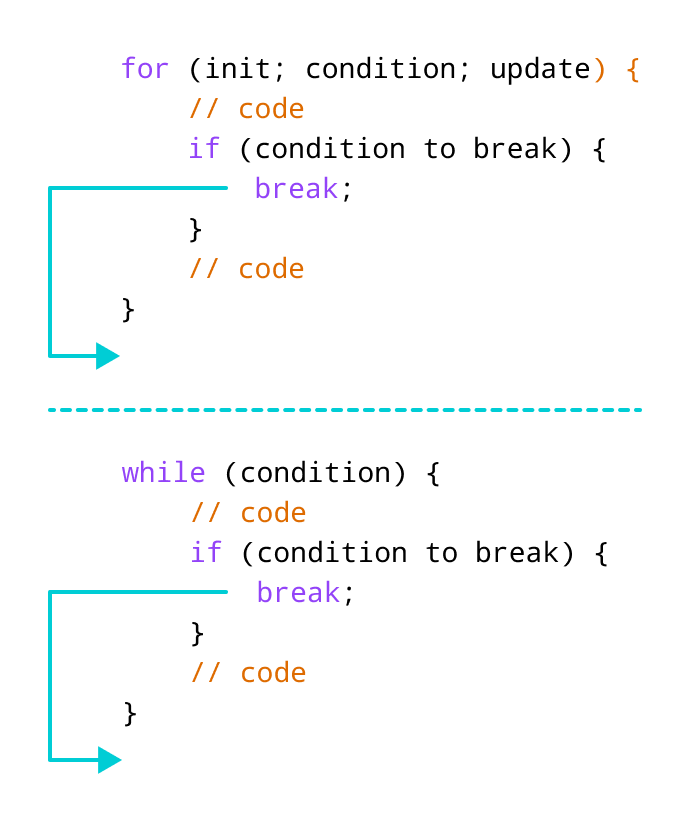# C++ break Statement

In this tutorial, we will learn about the break statement and its working in loops with the help of examples.

In C++, the `break` statement terminates the loop when it is encountered.

The syntax of the `break` statement is:

``break;``

Before you learn about the `break` statement, make sure you know about:

## Working of C++ break StatementWorking of break statement in C++

## Example 1: break with for loop

``````// program to print the value of i

#include <iostream>
using namespace std;

int main() {
for (int i = 1; i <= 5; i++) {
// break condition
if (i == 3) {
break;
}
cout << i << endl;
}

return 0;
}``````

Output

```1
2```

In the above program, the `for` loop is used to print the value of i in each iteration. Here, notice the code:

``````if (i == 3) {
break;
}``````

This means, when i is equal to 3, the `break` statement terminates the loop. Hence, the output doesn't include values greater than or equal to 3.

Note: The `break` statement is usually used with decision-making statements.

## Example 2: break with while loop

``````// program to find the sum of positive numbers
// if the user enters a negative numbers, break ends the loop
// the negative number entered is not added to sum

#include <iostream>
using namespace std;

int main() {
int number;
int sum = 0;

while (true) {
// take input from the user
cout << "Enter a number: ";
cin >> number;

// break condition
if (number < 0) {
break;
}

// add all positive numbers
sum += number;
}

// display the sum
cout << "The sum is " << sum << endl;

return 0;
}``````

Output

```Enter a number: 1
Enter a number: 2
Enter a number: 3
Enter a number: -5
The sum is 6. ```

In the above program, the user enters a number. The `while` loop is used to print the total sum of numbers entered by the user. Here, notice the code,

``````if(number < 0) {
break;
}``````

This means, when the user enters a negative number, the `break` statement terminates the loop and codes outside the loop are executed.

The `while` loop continues until the user enters a negative number.

## break with Nested loop

When `break` is used with nested loops, `break` terminates the inner loop. For example,

``````// using break statement inside
// nested for loop

#include <iostream>
using namespace std;

int main() {
int number;
int sum = 0;

// nested for loops

// first loop
for (int i = 1; i <= 3; i++) {
// second loop
for (int j = 1; j <= 3; j++) {
if (i == 2) {
break;
}
cout << "i = " << i << ", j = " << j << endl;
}
}

return 0;
}``````

Output

```i = 1, j = 1
i = 1, j = 2
i = 1, j = 3
i = 3, j = 1
i = 3, j = 2
i = 3, j = 3```

In the above program, the `break` statement is executed when `i == 2`. It terminates the inner loop, and the control flow of the program moves to the outer loop.

Hence, the value of i = 2 is never displayed in the output.

The `break` statement is also used with the `switch` statement. To learn more, visit C++ switch statement.